/

### Function Description

Returns the (sample) variance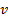of a series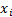(for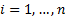), calculated as follows: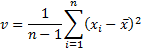(where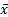is the mean of the series)

The equivalent function in Microsoft Excel is VAR.

If the observations are drawn from a normal distribution then the sample standard deviation is an unbiased estimate of the underlying population standard deviation.

Contents | Prev | Next